# Discrete Gabor Transform and Expansion (Advanced Signal Processing Toolkit)

LabVIEW 2014 Advanced Signal Processing Toolkit Help

Edition Date: June 2014

Part Number: 372656C-01

»View Product InfoDownload Help (Windows Only)

The discrete Gabor transform is an invertible, linear time-frequency transform. The discrete Gabor expansion is the inverse of the discrete Gabor transform. The output of the discrete Gabor transform is called the Gabor coefficients.

Characteristics of time-varying signals that are not obvious in the time domain or in the frequency domain alone can become clear in the time-frequency domain when you apply the discrete Gabor transform. After you extract the useful features of a signal in the time-frequency domain, you can apply the discrete Gabor expansion to obtain the time waveform with the extracted features. Similarly, after you suppress the useless components, like noise, in the time-frequency domain, you can apply the discrete Gabor expansion to obtain the time waveform with the noise suppressed.

In the following equation, the Gabor expansion represents a time-domain signal, s(i), as the linear combination of elementary functions hm,n(i):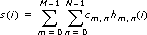where hm,n(i) is the time-frequency elementary function, Cm,n is the weight of hm,n(i), and Cm,n is the Gabor coefficients.

The Gabor transform computes the Gabor coefficients Cm,n for the signal s(i).

## Synthesis Window, Analysis Window, and Dual Window

Because the discrete Gabor transform is a special case of the STFT, you must consider the effects of the window characteristics and understand how the window length and type affect the time-frequency resolution of the time-frequency representation. The window used with the discrete Gabor transform is called the analysis window. The window used with the discrete Gabor expansion is called the synthesis window.

The following equation defines the time-shifted and frequency-modulated version, hm,n(i), of a window function, h(i):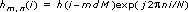where h(i) is the synthesis window, dM is time step, and N is frequency bins. Cm,n reveals how the signal behaves in the joint time-frequency domain around the time and the frequency centers of hm,n(i).

You can use the Gabor transform to obtain the Gabor coefficients Cm,n with the following equation:whereis the analysis window.and h(i) are a pair of dual functions because you use one for the Gabor transform and the other for the Gabor expansion, respectively. Usually, you specify the synthesis window h(i) first and then use the TFA Dual Window VI to compute the corresponding analysis window ().

To reconstruct the time-domain signal accurately from the Gabor time-frequency representation, you must use appropriate, complementary analysis and synthesis windows. You can exchange the analysis windows and the synthesis windows, meaning that you can use the synthesis windows for the Gabor transform and use the analysis windows for the Gabor expansion. Therefore, the analysis windows and the synthesis windows are called dual windows. Usually, you first specify the synthesis window, such as a Gaussian window. Given a synthesis window, you must use the TFA Dual Window VI to compute the corresponding analysis window. You also can use the Dual Window Express VI to design the dual windows for the discrete Gabor transform and the discrete Gabor expansion interactively.

## Oversampling Rate

You can consider hm,n(i) as the discrete atoms in the time-frequency domain as shown in the following grid: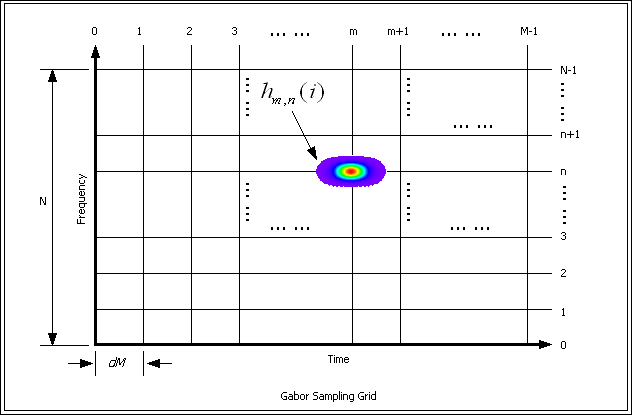The cross points in the grid are the time and the frequency centers of the elementary functions. The time and the frequency centers of hm,n(i) in normalized units are mdM and 2n/N, respectively.

You can consider the Gabor expansion as a way to resample the time-domain signal in the joint time-frequency domain. The time sampling interval in the joint time-frequency domain is dM. The frequency sampling interval in the joint time-frequency domain is 2/N.

The time centers and frequency centers of the elementary functions used in a particular Gabor transform application are discrete and form an equally sampled grid in the time-frequency domain, as shown in the previous figure. If the time-frequency center of a signal component is not aligned with the time-frequency sampling grid, some spectral energy from that signal component might spread erroneously to adjacent points in the time-frequency plane. This energy leakage can distort the resulting time-frequency spectrum and introduce confusing artifacts in the spectrum. Therefore, the discrete Gabor transform and the discrete Gabor expansion are not always the best way to analyze time-varying signals.

The number of sampling points in the time-frequency domain is M×N. The ratio of the total sampling points in the time-frequency domain and in the time domain, that is, MN/L, is called the oversampling rate, where L is the signal length. Because M = L/dM, the oversampling rate equals N/dM. The oversampling rate must be greater than or equal to 1 to reconstruct the time-domain signal from the time-frequency domain representation.

## Using the Discrete Gabor Transform and Discrete Gabor Expansion

The following figure shows the typical steps for creating applications using the discrete Gabor transform and the discrete Gabor expansion.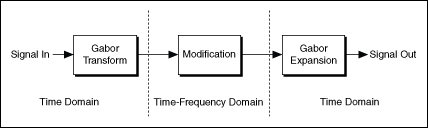To reduce noise or separate components of a time-varying signal, you first use the discrete Gabor transform to compute the time-frequency representation of a signal, modify the time-frequency representation of the signal, and then apply the discrete Gabor expansion to reconstruct the time-domain signal with the modified time-frequency representation.

Use the TFA Discrete Gabor Transform VI and the TFA Discrete Gabor Expansion VI to compute the Gabor transform and Gabor expansion.

Masking and thresholding are the most commonly used methods to modify a signal in the time-frequency domain. A mask defines which parts of the time-frequency representation remain and which parts to set to zero. The threshold defines which parts of the time-frequency representation with magnitudes greater than the threshold remain and which parts to set to zero. The mask and the threshold are similar to filters with time-dependent specifications. This kind of application commonly is called time-varying filtering.

Use the TFA Time Varying Filter VI and the Time Varying Filter (Thresholding) Express VI to perform time-varying filtering interactively, such as in noise reduction and feature extraction applications. Many other methods exist to modify the time-frequency representation, for example, setting the phase of the time-frequency representation to zero or setting the magnitude of the time-frequency representation to unity.

The following figure shows an example of a noisy signal that contains three chirps.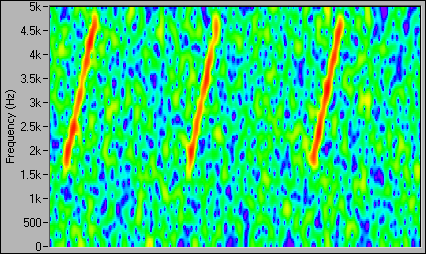The color corresponds to the magnitude of the Gabor coefficients from the discrete Gabor transform. The color spectrum from red to blue corresponds to the magnitude from maximum to minimum. The Gabor coefficients that correspond to the chirps have a higher magnitude and are concentrated in a relatively small region in the time-frequency domain. The Gabor coefficients that correspond to the noise have a lower magnitude and are spread over the entire time-frequency domain. By applying a thresholding operation, you can preserve the Gabor coefficients that correspond to the chirps and reduce to zero the Gabor coefficients that correspond to noise. The Time Varying Filter (Thresholding) Express VI reconstructs the denoised chirps from these modified Gabor coefficients by using the discrete Gabor expansion, as shown in the following figure.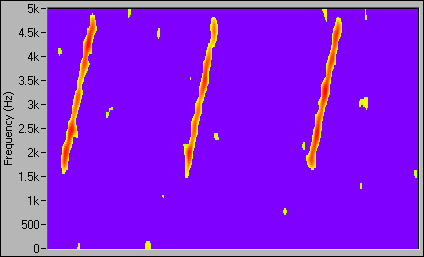Refer to the Offline Noise Reduction with Time Varying Filter VI in the labview\examples\Time Frequency Analysis\TFAApplications directory for an example of using the Time Varying Filter (Thresholding) Express VI.